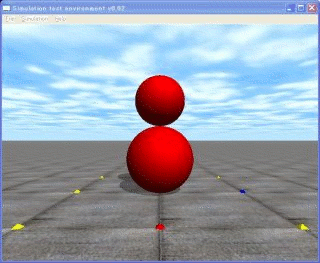# ODE Tutorial 17: Offset the Center of Gravity

Most of this page was translated from http://demura.net/archives/9ode by Babel Fish Translation.
Sorry for strange and funny English from “the Chinese room“.This is 17th article about ODE (Open Dynamics Engine) Tutorial. ODE is an open source physics libary, and it is widely used  in various game software, and simulators in research.

This time it explains concerning the method of moving the position of COG (center of gravity). API, dGeomSetOffsetPosition was introduced from ODE0.6, shifting the position of center of gravity extremelysimply in comparison withthe former method of usingthe Geometry Transform object whichlast year is introduced with ODE lecture reached the point where it can set.

• API about offset the positon of geometry
Void dGeomSetOffsetPosition (dGeomID and dReal x, dReal y and dReal z);
(X, just y and z) shifting the position of dGeomID from the position of body, it sets. Before using this API, geometry must be correlated with the body. In other words, it is necessary for dGeomSetBody to be first called.

So, the tumbler in the upper figure is introduced as a sample program.Please download the source code from here.With this example, it is suitable to the body of the small priest sphere (mass 10kg and radius 0.4m) from the center of sphere under 0.4m to set center of gravity, because as for mass of the sphere which is suitable to the head there is only 1g, this small priest by all means rises. Concretely, with dBodySetPosition from the center of sphere radius amount (0.4m) shifting balance station under, it sets, that was shifted the quantity which (the offset, here dz) it has set with dGeomSetOffsetPosition.

When the f key and the j key are pushed from the keyboard, to the tumbler it can add to left and right direction the power of 100N, but being to be a restitutive force, it rises, it requires you can verify.

```// koboshi2.cpp by Kosei Demura 2007-1-20
#include <ode/ode.h>
#include <drawstuff/drawstuff.h>;
#ifdef dDOUBLE
#define dsDrawSphere dsDrawSphereD
#endif
#define MAX_CONTACTS 4
static dWorldID world;
static dSpaceID space;
static dGeomID  ground;
static dJointGroupID contactgroup;
dsFunctions fn;
typedef struct {
dBodyID body;
dGeomID geom;
dReal   length;
dReal   mass;

dJointID joint;　　　　　//  a fixed joint between a head and a torso
static void nearCallback (void *data, dGeomID o1, dGeomID o2);
{
int i;
// exit without doing anything if the two bodies are connected by a joint
dBodyID b1 = dGeomGetBody(o1);
dBodyID b2 = dGeomGetBody(o2);
if (b1 && b2 && dAreConnectedExcluding (b1,b2,dJointTypeContact)) return;
dContact contact[MAX_CONTACTS];   // up to MAX_CONTACTS contacts per box-box

for (i=0; i < N; i++) {
contact[i].surface.mode = dContactBounce | dContactSoftCFM;
contact[i].surface.mu = 1.0;                   // Coefficient of friction
contact[i].surface.bounce = 0.01;          //  bounce parameter
contact[i].surface.bounce_vel = 0.01;   //  minimum incoming velocity necessary for  bounce
contact[i].surface.soft_cfm = 0.00001;
}

if (int numc = dCollide (o1,o2,MAX_CONTACTS,&contact.geom,sizeof(dContact))) {
for (i=0; i < numc; i++) {
dJointID c = dJointCreateContact (world,contactgroup,contact+i);
dJointAttach (c,b1,b2);
}
}
}

static void drawSphere(dGeomID g)
{
if (!g) return;
const dReal *pos = dGeomGetPosition(g);
const dReal *R = dGeomGetRotation(g);
}

static void simLoop (int pause)
{
dSpaceCollide(space,0,&nearCallback);
dWorldStep(world,0.01);
dJointGroupEmpty(contactgroup);
dsSetColor(1.0,0.0,0.0);
drawSphere(torso.geom);
dsSetColor(1.0, 0.0, 0.0);
}

void start()
{
static float xyz = {   3.5,0.0,1.0};
static float hpr  = {-180.0,0.0,0.0};
dsSetSphereQuality(3);
dsSetViewpoint(xyz,hpr);
}

// Create an object
void createDaruma()
{
dMass m,m1;
dReal x0 = 0.0, y0 = 0.0, z0 = 0.4;
dReal dx = 0.0, dy = 0.0, dz = 0.4;  // offset
// torso: offset the center of gravity
torso.body   = dBodyCreate(world);
torso.mass   = 10.0;　//  mass

dMassSetZero(&m);
dBodySetMass(torso.body, &m);
dGeomSetBody(torso.geom,torso.body);

// Set the COG under the radius of the torso (sphere)
dBodySetPosition(torso.body, x0, y0, z0 - dz);

//This way, in order geometry of the body (form) to be buried in the land, it sets with respect to radius amount of sphere. Center of gravity of sphere moves under radius amount due to this movement, the body has restitutive force.
dGeomSetOffsetPosition(torso.geom, 0, 0, dz);

dMassSetZero(&m1);

//  fixed joint (between the head and the torso)
joint = dJointCreateFixed(world, 0);
dJointSetFixed(joint);
}

void command(int cmd)
{
switch(cmd) {
case ‘f’:　// When the f key is pushed, the power of 100N is added to forward of x axis
case ‘j’:　// When  the j key is pushed, -100N power is added to forward of x axis
}
}

void  setDrawStuff() {
fn.version = DS_VERSION;
fn.start   = &start;
fn.step    = &simLoop;
fn.command = &command;
fn.stop    = NULL;
fn.path_to_textures = "../../drawstuff/textures";
}

int main(int argc, char **argv)
{
setDrawStuff();    // Set of drawing function
world = dWorldCreate();　// create a dynamic world
space = dHashSpaceCreate(0);　// 　create a collision space
contactgroup = dJointGroupCreate(0);　// for collision calculation
dWorldSetGravity(world,0,0,-9.8);　// set a gravity of the dynamic world
ground = dCreatePlane(space,0,0,1,0);　//  set a ground
createDaruma();　// create a Daruma
dsSimulationLoop (argc,argv,640,480,&fn);　//  simulation loop
dSpaceDestroy(space);     //  destory the collision space
dWorldDestroy (world); 　//  destroy the dynamic world<
return 0;
}
```
english
スポンサーリンク
demura.netをフォローするdemura.net

タイトルとURLをコピーしました